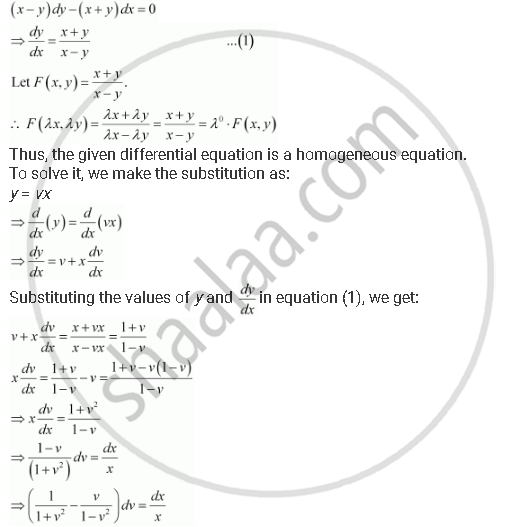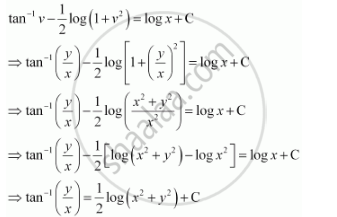Share

# Show that the Given Differential Equation is Homogeneous and Solve Each of Them (X – Y) Dy – (X + Y) Dx = 0 - CBSE (Commerce) Class 12 - Mathematics

ConceptMethods of Solving First Order, First Degree Differential Equations Homogeneous Differential Equations

#### Question

Show that the given differential equation is homogeneous and solve each of them

(x – y) dy – (x + y) dx = 0

#### Solution

The given differential equation is:Integrating both sides, we get:This is the required solution of the given differential equation.

Is there an error in this question or solution?

#### APPEARS IN

NCERT Solution for Mathematics Textbook for Class 12 (2018 to Current)
Chapter 9: Differential Equations
Q: 3 | Page no. 406
Solution Show that the Given Differential Equation is Homogeneous and Solve Each of Them (X – Y) Dy – (X + Y) Dx = 0 Concept: Methods of Solving First Order, First Degree Differential Equations - Homogeneous Differential Equations.
S